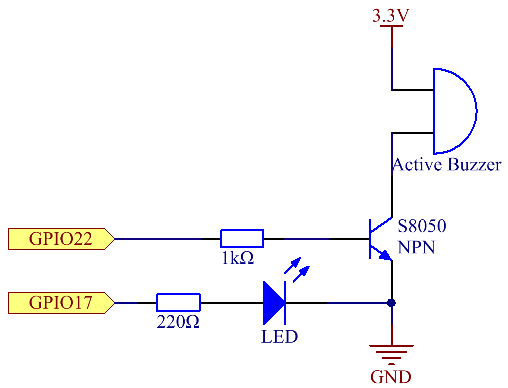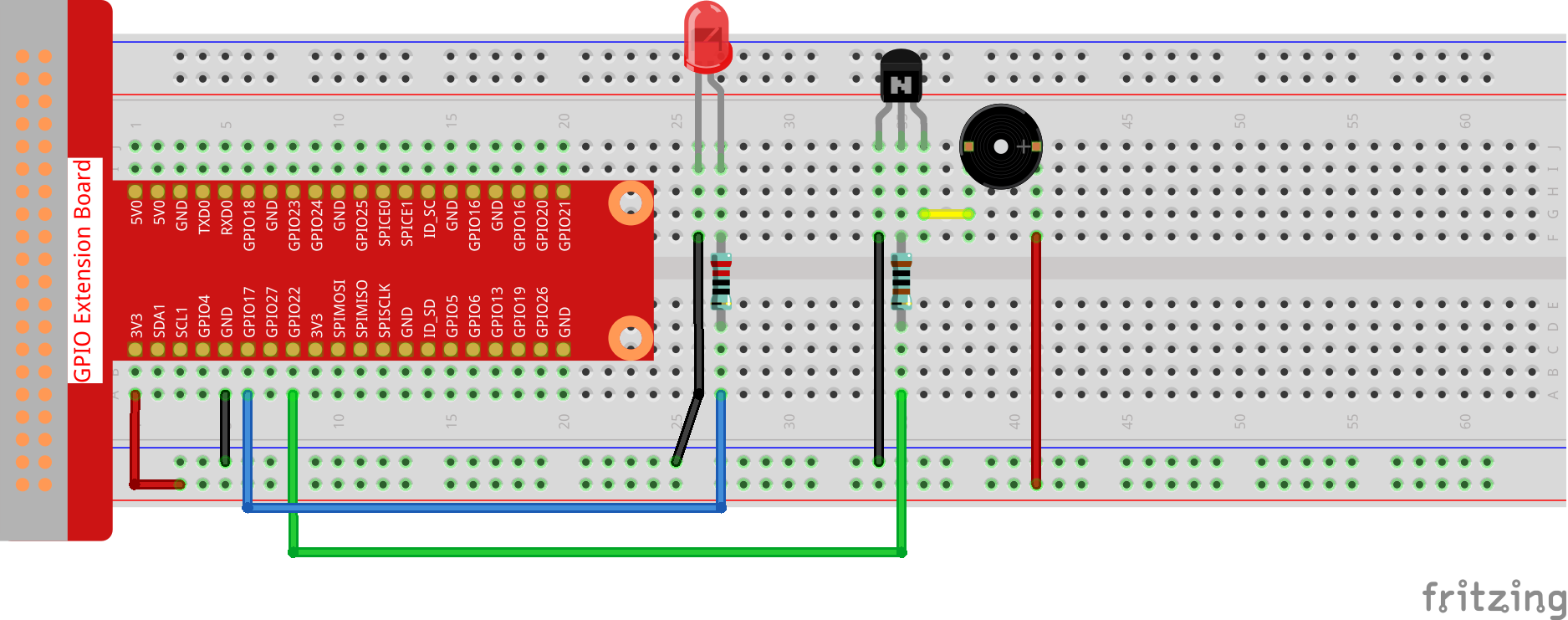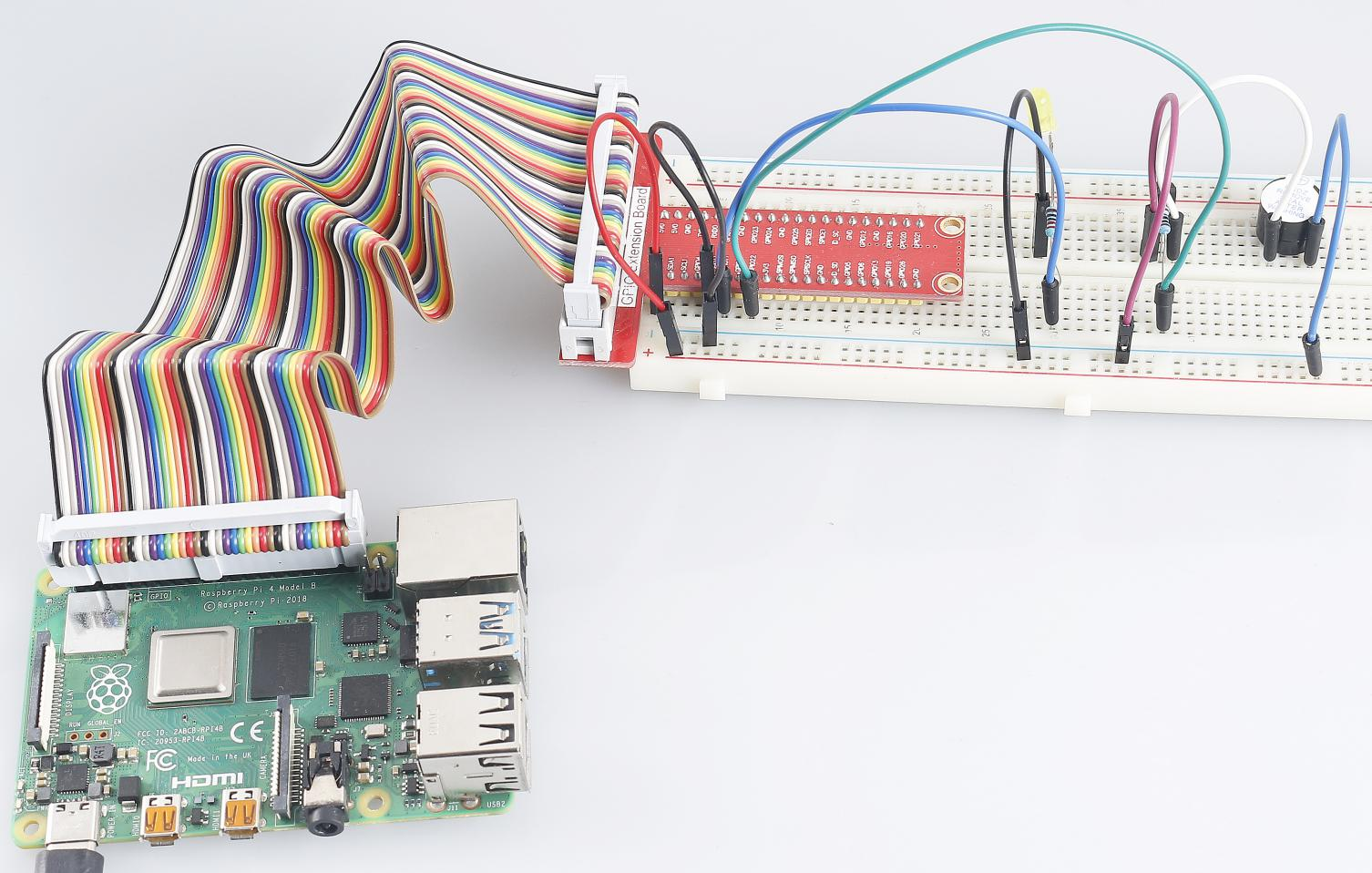# 3.1.11 Morse Code Generator¶

## Introduction¶

In this lesson, we’ll make a Morse code generator, where you type in a series of English letters in the Raspberry Pi to make it appear as Morse code.

## Components¶## Schematic Diagram¶

 T-Board Name physical wiringPi BCM GPIO17 Pin 11 0 17 GPIO22 Pin 15 3 22## Experimental Procedures¶

Step 1: Build the circuit. (Pay attention to poles of the buzzer: The one with + label is the positive pole and the other is the negative.)### For C Language Users¶

Step 2: Open the code file.

```cd ~/davinci-kit-for-raspberry-pi/c/3.1.11/
```

Step 3: Compile the code.

```gcc 3.1.11_MorseCodeGenerator.c -lwiringPi
```

Step 4: Run the executable file above.

```sudo ./a.out
```

After the program runs, type a series of characters, and the buzzer and the LED will send the corresponding Morse code signals.

Note

If it does not work after running, or there is an error prompt: "wiringPi.h: No such file or directory", please refer to C code is not working?.

Code Explanation

```struct MORSE{
char word;
unsigned char *code;
};

struct MORSE morseDict[]=
{
{'A',"01"}, {'B',"1000"}, {'C',"1010"}, {'D',"100"}, {'E',"0"},
{'F',"0010"}, {'G',"110"}, {'H',"0000"}, {'I',"00"}, {'J',"0111"},
{'K',"101"}, {'L',"0100"}, {'M',"11"}, {'N',"10"}, {'O',"111"},
{'P',"0110"}, {'Q',"1101"}, {'R',"010"}, {'S',"000"}, {'T',"1"},
{'U',"001"}, {'V',"0001"}, {'W',"011"}, {'X',"1001"}, {'Y',"1011"},
{'Z',"1100"},{'1',"01111"}, {'2',"00111"}, {'3',"00011"}, {'4',"00001"},
{'5',"00000"},{'6',"10000"}, {'7',"11000"}, {'8',"11100"}, {'9',"11110"},
{'0',"11111"},{'?',"001100"}, {'/',"10010"}, {',',"110011"}, {'.',"010101"},
{';',"101010"},{'!',"101011"}, {'@',"011010"}, {':',"111000"}
};
```

This structure MORSE is the dictionary of the Morse code, containing characters A-Z, numbers 0-9 and marks "?" "/" ":" "," "." ";" "!" "@" .

```char *lookup(char key,struct MORSE *dict,int length)
{
for (int i=0;i<length;i++)
{
if(dict[i].word==key){
return dict[i].code;
}
}
}
```

The function lookup() works by "checking the dictionary". Define a key, search the same words as key in the structure morseDict and return the corresponding information— "code" of the certain word.

```void on(){
digitalWrite(ALedPin,HIGH);
digitalWrite(BeepPin,HIGH);
}
```

Create a function on() to start the buzzer and the LED.

```void off(){
digitalWrite(ALedPin,LOW);
digitalWrite(BeepPin,LOW);
}
```

The function off() turns off the buzzer and the LED.

```void beep(int dt){
on();
delay(dt);
off();
delay(dt);
}
```

Define a function beep() to make the buzzer and the LED emit sounds and blink in a certain interval of dt.

```void morsecode(char *code){
int pause = 250;
char *point = NULL;
int length = sizeof(morseDict)/sizeof(morseDict);
for (int i=0;i<strlen(code);i++)
{
point=lookup(code[i],morseDict,length);
for (int j=0;j<strlen(point);j++){
if (point[j]=='0')
{
beep(pause/2);
}else if(point[j]=='1')
{
beep(pause);
}
delay(pause);
}
}
}
```

The function morsecode() is used to process the Morse code of input characters by making the "1" of the code keep emitting sounds or lights and the "0"shortly emit sounds or lights, ex., input "SOS", and there will be a signal containing three short three long and then three short segments " · · · - - - · · · ".

```int toupper(int c)
{
if ((c >= 'a') && (c <= 'z'))
return c + ('A' - 'a');
return c;
}
char *strupr(char *str)
{
char *orign=str;
for (; *str!='\0'; str++)
*str = toupper(*str);
return orign;
}
```

Before coding, you need to unify the letters into capital letters.

```void main(){
setup();
char *code;
int length=8;
code = (char*)malloc(sizeof(char)*length);
while (1){
delay(100);
scanf("%s",code);
code=strupr(code);
printf("%s\n",code);
delay(100);
morsecode(code);
}
}
```

When you type the relevant characters with the keyboard, code=strupr(code) will convert the input letters to their capital form.

Printf() then prints the clear text on the computer screen, and the morsecod() function causes the buzzer and the LED to emit Morse code.

Note that the length of the input character mustn’t exceed the length (can be revised).

### For Python Language Users¶

Step 2: Open the code file.

```cd ~/davinci-kit-for-raspberry-pi/python
```

Step 3: Run.

```sudo python3 3.1.11_MorseCodeGenerator.py
```

After the program runs, type a series of characters, and the buzzer and the LED will send the corresponding Morse code signals.

Code

Note

You can Modify/Reset/Copy/Run/Stop the code below. But before that, you need to go to source code path like `davinci-kit-for-raspberry-pi/python`.

```import RPi.GPIO as GPIO
import time

BeepPin=22
ALedPin=17

MORSECODE = {
'A':'01', 'B':'1000', 'C':'1010', 'D':'100', 'E':'0', 'F':'0010', 'G':'110',
'H':'0000', 'I':'00', 'J':'0111', 'K':'101', 'L':'0100', 'M':'11', 'N':'10',
'O':'111', 'P':'0110', 'Q':'1101', 'R':'010', 'S':'000', 'T':'1',
'U':'001', 'V':'0001', 'W':'011', 'X':'1001', 'Y':'1011', 'Z':'1100',
'1':'01111', '2':'00111', '3':'00011', '4':'00001', '5':'00000',
'6':'10000', '7':'11000', '8':'11100', '9':'11110', '0':'11111',
'?':'001100', '/':'10010', ',':'110011', '.':'010101', ';':'101010',
'!':'101011', '@':'011010', ':':'111000',
}

def setup():
GPIO.setmode(GPIO.BCM)
GPIO.setup(BeepPin, GPIO.OUT, initial=GPIO.LOW)
GPIO.setup(ALedPin,GPIO.OUT,initial=GPIO.LOW)

def on():
GPIO.output(BeepPin, 1)
GPIO.output(ALedPin, 1)

def off():
GPIO.output(BeepPin, 0)
GPIO.output(ALedPin, 0)

def beep(dt):       # dt for delay time.
on()
time.sleep(dt)
off()
time.sleep(dt)

def morsecode(code):
pause = 0.25
for letter in code:
for tap in MORSECODE[letter]:
if tap == '0':
beep(pause/2)
if tap == '1':
beep(pause)
time.sleep(pause)

def main():
while True:
code = code.upper()
print(code)
morsecode(code)

def destroy():
print("")
GPIO.output(BeepPin, GPIO.LOW)
GPIO.output(ALedPin, GPIO.LOW)
GPIO.cleanup()

if __name__ == '__main__':
setup()
try:
main()
except KeyboardInterrupt:
destroy()
```

Code Explanation

```MORSECODE = {
'A':'01', 'B':'1000', 'C':'1010', 'D':'100', 'E':'0', 'F':'0010', 'G':'110',
'H':'0000', 'I':'00', 'J':'0111', 'K':'101', 'L':'0100', 'M':'11', 'N':'10',
'O':'111', 'P':'0110', 'Q':'1101', 'R':'010', 'S':'000', 'T':'1',
'U':'001', 'V':'0001', 'W':'011', 'X':'1001', 'Y':'1011', 'Z':'1100',
'1':'01111', '2':'00111', '3':'00011', '4':'00001', '5':'00000',
'6':'10000', '7':'11000', '8':'11100', '9':'11110', '0':'11111',
'?':'001100', '/':'10010', ',':'110011', '.':'010101', ';':'101010',
'!':'101011', '@':'011010', ':':'111000',
}
```

This structure MORSE is the dictionary of the Morse code, containing characters A-Z, numbers 0-9 and marks "?" "/" ":" "," "." ";" "!" "@" .

```def on():
GPIO.output(BeepPin, 1)
GPIO.output(ALedPin, 1)
```

The function on() starts the buzzer and the LED.

```def off():
GPIO.output(BeepPin, 0)
GPIO.output(ALedPin, 0)
```

The function off() is used to turn off the buzzer and the LED.

```def beep(dt):   # x for dalay time.
on()
time.sleep(dt)
off()
time.sleep(dt)
```

Define a function beep() to make the buzzer and the LED emit sounds and blink in a certain interval of dt.

```def morsecode(code):
pause = 0.25
for letter in code:
for tap in MORSECODE[letter]:
if tap == '0':
beep(pause/2)
if tap == '1':
beep(pause)
time.sleep(pause)
```

The function morsecode() is used to process the Morse code of input characters by making the "1" of the code keep emitting sounds or lights and the "0"shortly emit sounds or lights, ex., input "SOS", and there will be a signal containing three short three long and then three short segments " · · · - - - · · · ".

```def main():
while True: